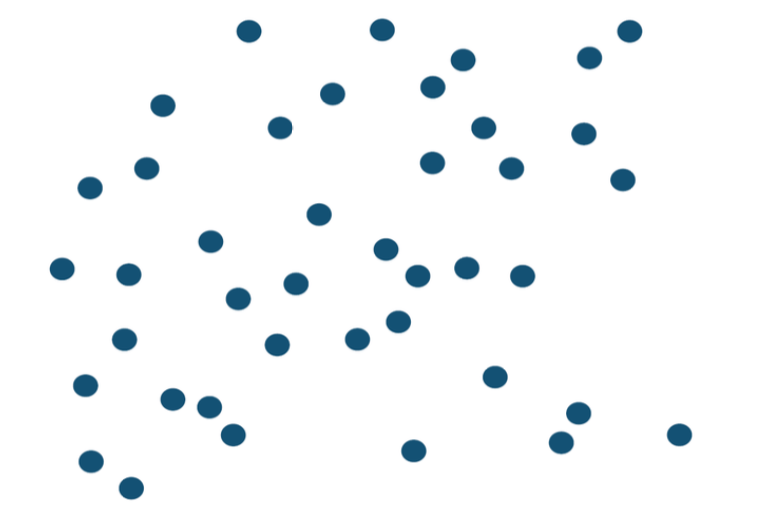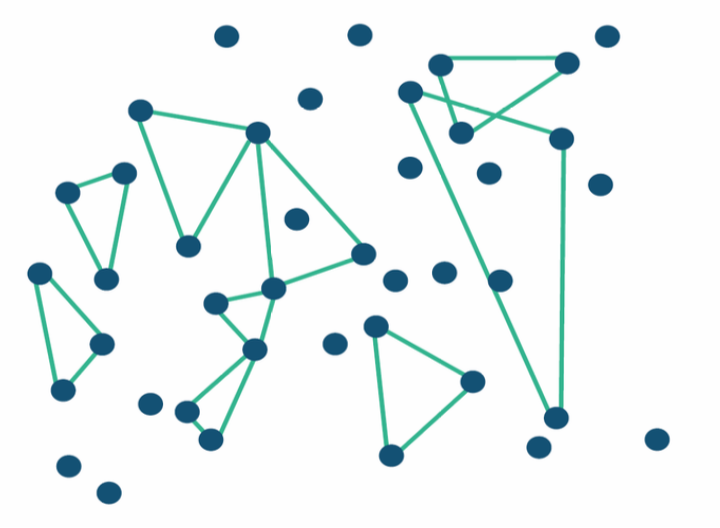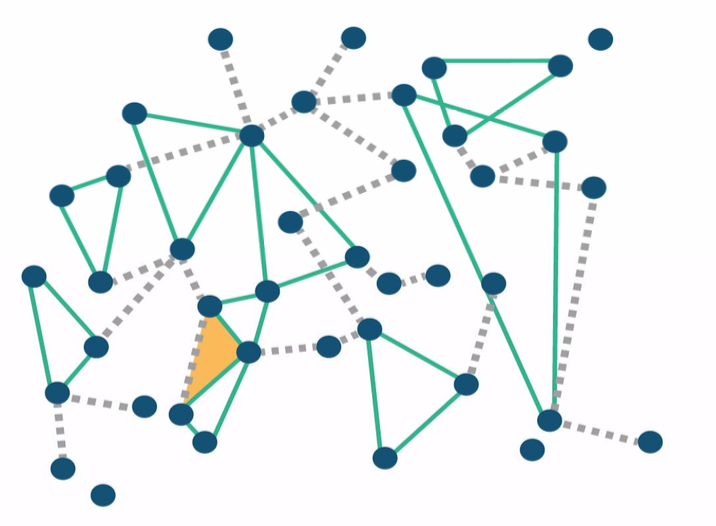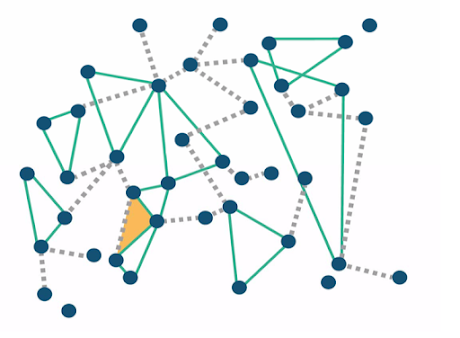# SUGMs¶

• Subgraph Generation Models
• Subgraphs are generated, network is by-product

what the idea here is again we're going to be looking at, at ways to easily incorporate dependencies and networks and think about different kinds of sub graphs that might be forming and allow those to be generated and try and estimate how they're being generated. And the idea of SUGMs the, the, the terminology is quite simple. It's from subgraph generation model. So we think of subgraphs as being generated. Links, triangles small stars etcetera. Those are the things that are going to be generated, and then the network is a byproduct, right? So what's happening is people are forming different types of relationships, different kinds of cliques, and the network bubbles up from that.

• Nature/people form $S_j$ subnetworks of type j each indepedently with probability $p_j$
• May intersect and overlap, sometimes people form links, they also form a clique, so I end up partnering with somebody but I also form groups
• We observe resulting network, infer the $p_j's$

# Examples¶# Links Form: Incidental Triangle¶# We see¶## Relations: SERGMs/SUGMs¶

• Can we view SUGMs as SERGMs?
• Yes, and it motivates specific K's
• The true counts of exactly how many triangles are formed and how many links are formed aren't exactly observable.
• However, can get close to estimating them and observing them when things are sparse
• So, sparse SUGMs define easily estimated class of SERGMs...

# Theorem: SUGMs and SERGMs¶

Consider a SUGM with parameter $p_j$ and let S be the true counts of subgraphs

Then $Pr(S) = \frac{K(S)exp(\beta S)}{\sum_{S'}K(S')exp(\beta S')}$

$\beta_j = log(P_j / (1 - p_j))$ and $K^n(S) = \prod_j \binom{S_j}{\bar S_j^n}$

# Sparse: Few Incidental Triangles¶